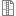# Nonmanifold model stitch (sew) bug

Hi all,

I have a simple iges file. It contains only two faces. When I try to stitch them together using non-manifold model, it can't be stitched, but it works well under manifold mode. I traced the code and I think it's a bug in BRepBuilderAPI_Sewing::MergedNearestEdges.

// Find the longest edge CCI60011
Standard_Integer i, indRef = 1;
if (myNonmanifold) {
Standard_Real lenRef = 0.;
for (i = 1; i Standard_Real f, l;
Handle(Geom_Curve) c3d = BRep_Tool::Curve(TopoDS::Edge(seqEdges(i)), f, l);
Standard_Real len = GCPnts_AbscissaPoint::Length(cAdapt, f, l);
if (len > lenRef) { indRef = i; lenRef = len; }
}
if (indRef != 1) {
TopoDS_Shape longEdge = seqEdges(indRef);
seqEdges(indRef) = seqEdges(1);
seqEdges(1) = longEdge;
}
}

This code try to sort the candidate edges according to the length under non-manifold mode.
However, the following codes will never get the correct merged edges if the first candidate edge is not a real edge which should be merged.
Here, I comment this code, but I suspect it will cause some other bugs according to the hint "// Find the longest edge CCI60011".

Any suggestion is welcome. Thanks in advance.

-Ding

Attachments:stitch.zip